# 数字图像处理(19): 边缘检测算子(Roberts算子、Prewitt算子、Sobel算子 和 Laplacian算子)1 边缘检测的基本原理

2 边缘检测算子分类

3 梯度

3.1 图像梯度

3.2 梯度算子

4 Roberts 算子

4.1 基本原理

4.2 代码示例

5 Prewitt 算子

5.1 基本原理

5.2 代码示例

6 Sobel 算子

6.1 基本原理

6.2 代码示例

7 Laplacian 算子

7.1 基本原理

7.2 代码示例

8 小结

8.1 各类算子实验比较

8.2 各类算子的优缺点

（1）一阶导数的边缘算子

（2）二阶导数的边缘算子

（3）其他边缘算子

# 3 梯度

## 3.1 图像梯度

## 3.2 梯度算子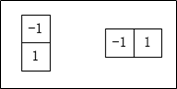# 4 Roberts 算子

## 4.1 基本原理

Roberts算子又称为交叉微分算法，它是基于交叉差分的梯度算法，通过局部差分计算检测边缘线条。常用来处理具有陡峭的低噪声图像，当图像边缘接近于正45度或负45度时，该算法处理效果更理想。其缺点是对边缘的定位不太准确，提取的边缘线条较粗。

Roberts算子的模板分为水平方向和垂直方向，如下式所示，从其模板可以看出，Roberts算子能较好的增强正负45度的图像边缘。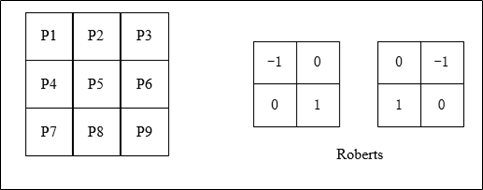## 4.2 代码示例

dst = filter2D(src, ddepth, kernel[, dst[, anchor[, delta[, borderType]]]])

src 表示输入图像；

dst 表示输出的边缘图，其大小和通道数与输入图像相同；

ddepth 表示目标图像所需的深度；

kernel 表示卷积核，一个单通道浮点型矩阵；

anchor 表示内核的基准点，其默认值为 (-1，-1)，位于中心位置；

delta 表示在储存目标图像前可选的添加到像素的值，默认值为0；

borderType 表示边框模式。

# -*- coding: utf-8 -*-
import cv2
import numpy as np
import matplotlib.pyplot as plt

# 读取图像
img_RGB = cv2.cvtColor(img, cv2.COLOR_BGR2RGB) #转成RGB 方便后面显示

# 灰度化处理图像
grayImage = cv2.cvtColor(img, cv2.COLOR_BGR2GRAY)

# Roberts算子
kernelx = np.array([[-1, 0], [0, 1]], dtype=int)
kernely = np.array([[0, -1], [1, 0]], dtype=int)
x = cv2.filter2D(grayImage, cv2.CV_16S, kernelx)
y = cv2.filter2D(grayImage, cv2.CV_16S, kernely)
# 转uint8
absX = cv2.convertScaleAbs(x)
absY = cv2.convertScaleAbs(y)
Roberts = cv2.addWeighted(absX, 0.5, absY, 0.5, 0)

# 用来正常显示中文标签
plt.rcParams['font.sans-serif'] = ['SimHei']

# 显示图形
# titles = [u'原始图像', u'Roberts算子']
# images = [img_RGB, Roberts]
# for i in range(2):
#     plt.subplot(1, 2, i + 1), plt.imshow(images[i], 'gray')
#     plt.title(titles[i])
#     plt.xticks([]), plt.yticks([])

# plt.show()

# 显示图形
plt.subplot(121),plt.imshow(img_RGB),plt.title('原始图像'), plt.axis('off') #坐标轴关闭
plt.subplot(122),plt.imshow(Roberts, cmap=plt.cm.gray ),plt.title('Roberts算子'), plt.axis('off')
plt.show()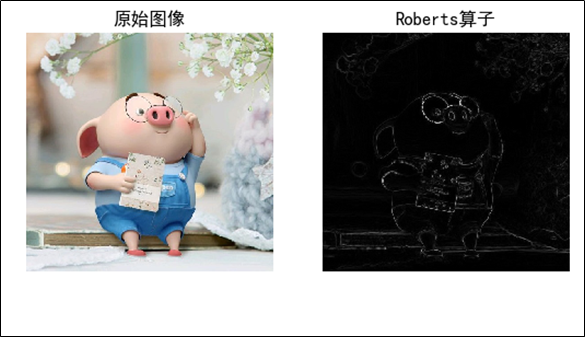# 5 Prewitt 算子

## 5.1 基本原理

Prewitt算子是一种图像边缘检测的微分算子，其原理是利用特定区域内像素灰度值产生的差分实现边缘检测。由于Prewitt算子采用 33 模板对区域内的像素值进行计算，而Robert算子的模板为 22，故Prewitt算子的边缘检测结果在水平方向和垂直方向均比Robert算子更加明显。Prewitt算子适合用来识别噪声较多、灰度渐变的图像，其计算公式如下所示：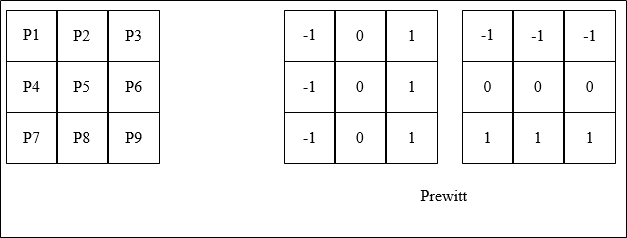## 5.2 代码示例

dst = filter2D(src, ddepth, kernel[, dst[, anchor[, delta[, borderType]]]])

src 表示输入图像；

dst 表示输出的边缘图，其大小和通道数与输入图像相同；

ddepth 表示目标图像所需的深度；

kernel 表示卷积核，一个单通道浮点型矩阵；

anchor 表示内核的基准点，其默认值为(-1，-1)，位于中心位置；

delta 表示在储存目标图像前可选的添加到像素的值，默认值为0；

borderType 表示边框模式。

# -*- coding: utf-8 -*-
import cv2
import numpy as np
import matplotlib.pyplot as plt

# 读取图像
img_RGB = cv2.cvtColor(img, cv2.COLOR_BGR2RGB)

# 灰度化处理图像
grayImage = cv2.cvtColor(img, cv2.COLOR_BGR2GRAY)

# Prewitt算子
kernelx = np.array([[1, 1, 1], [0, 0, 0], [-1, -1, -1]], dtype=int)
kernely = np.array([[-1, 0, 1], [-1, 0, 1], [-1, 0, 1]], dtype=int)
x = cv2.filter2D(grayImage, cv2.CV_16S, kernelx)
y = cv2.filter2D(grayImage, cv2.CV_16S, kernely)
# 转uint8
absX = cv2.convertScaleAbs(x)
absY = cv2.convertScaleAbs(y)
Prewitt = cv2.addWeighted(absX, 0.5, absY, 0.5, 0)

# 用来正常显示中文标签
plt.rcParams['font.sans-serif'] = ['SimHei']

# 显示图形
# titles = [u'原始图像', u'Prewitt算子']
# images = [img_RGB, Prewitt]
# for i in range(2):
#     plt.subplot(1, 2, i + 1), plt.imshow(images[i], 'gray')
#     plt.title(titles[i])
#     plt.xticks([]), plt.yticks([])
# plt.show()

# 显示图形
plt.subplot(121),plt.imshow(img_RGB),plt.title('原始图像'), plt.axis('off') #坐标轴关闭
plt.subplot(122),plt.imshow(Prewitt, cmap=plt.cm.gray ),plt.title('Prewitt算子'), plt.axis('off')
plt.show()# 6 Sobel 算子

## 6.1 基本原理

Sobel算子是一种用于边缘检测的离散微分算子，它结合了高斯平滑和微分求导。该算子用于计算图像明暗程度近似值，根据图像边缘旁边明暗程度把该区域内超过某个数的特定点记为边缘。Sobel算子在Prewitt算子的基础上增加了权重的概念，认为相邻点的距离远近对当前像素点的影响是不同的，距离越近的像素点对应当前像素的影响越大，从而实现图像锐化并突出边缘轮廓。

Sobel算子根据像素点上下、左右邻点灰度加权差，在边缘处达到极值这一现象检测边缘。对噪声具有平滑作用，提供较为精确的边缘方向信息。因为Sobel算子结合了高斯平滑和微分求导（分化），因此结果会具有更多的抗噪性，当对精度要求不是很高时，Sobel算子是一种较为常用的边缘检测方法。

Sobel算子的边缘定位更准确，常用于噪声较多、灰度渐变的图像。其算法模板如下面的公式所示，其中  表示水平方向， 表示垂直方向。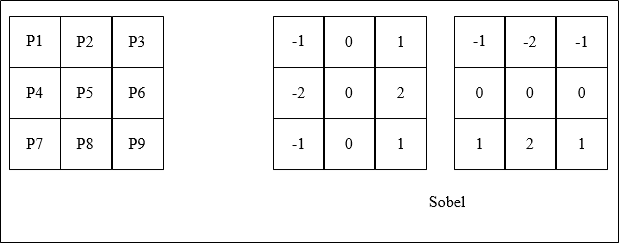## 6.2 代码示例

Sobel() 函数用法如下所示：

dst = Sobel(src, ddepth, dx, dy[, dst[, ksize[, scale[, delta[, borderType]]]]])

src 表示输入图像；

dst 表示输出的边缘图，其大小和通道数与输入图像相同；

ddepth 表示目标图像所需的深度，针对不同的输入图像，输出目标图像有不同的深度；

dx 表示  方向上的差分阶数，取值1或 0；

dy 表示  方向上的差分阶数，取值1或0；

ksize 表示Sobel算子的大小，其值必须是正数奇数

scale 表示缩放导数的比例常数，默认情况下没有伸缩系数；

delta 表示将结果存入目标图像之前，添加到结果中的可选增量值；

borderType 表示边框模式，更多详细信息查阅BorderTypes。

：在进行Sobel算子处理之后，还需要调用 convertScaleAbs() 函数计算绝对值，并将图像转换为8位图进行显示。其函数用法如下所示：

dst = convertScaleAbs(src[, dst[, alpha[, beta]]])

src 表示原数组；

dst 表示输出数组，深度为8位；

alpha 表示比例因子；

beta 表示原数组元素按比例缩放后添加的值。

# -*- coding: utf-8 -*-
import cv2
import numpy as np
import matplotlib.pyplot as plt

# 读取图像
img_RGB = cv2.cvtColor(img, cv2.COLOR_BGR2RGB)

# 灰度化处理图像
grayImage = cv2.cvtColor(img, cv2.COLOR_BGR2GRAY)

# Sobel算子
x = cv2.Sobel(grayImage, cv2.CV_16S, 1, 0)  # 对x求一阶导
y = cv2.Sobel(grayImage, cv2.CV_16S, 0, 1)  # 对y求一阶导
absX = cv2.convertScaleAbs(x)
absY = cv2.convertScaleAbs(y)
Sobel = cv2.addWeighted(absX, 0.5, absY, 0.5, 0)

# 用来正常显示中文标签
plt.rcParams['font.sans-serif'] = ['SimHei']

# # 显示图形
# titles = [u'原始图像', u'Sobel算子']
# images = [lenna_img, Sobel]
# for i in xrange(2):
#     plt.subplot(1, 2, i + 1), plt.imshow(images[i], 'gray')
#     plt.title(titles[i])
#     plt.xticks([]), plt.yticks([])
# plt.show()

# 显示图形
plt.subplot(121),plt.imshow(img_RGB),plt.title('原始图像'), plt.axis('off') #坐标轴关闭
plt.subplot(122),plt.imshow(Sobel, cmap=plt.cm.gray ),plt.title('Sobel算子'), plt.axis('off')
plt.show()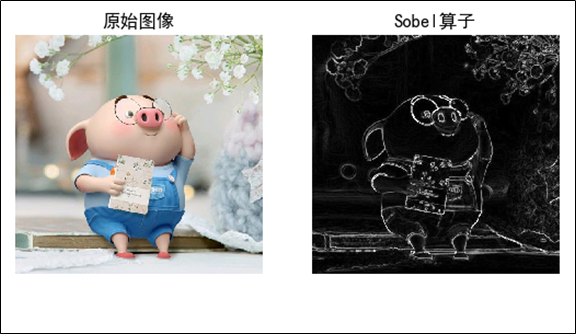# 7 Laplacian 算子

## 7.1 基本原理

1）判断图像中心像素灰度值与它周围其他像素的灰度值，如果中心像素的灰度更高，则提升中心像素的灰度；反之降低中心像素的灰度，从而实现图像锐化操作；

2）在算法实现过程中，Laplacian算子通过对邻域中心像素的四方向或八方向求梯度，再将梯度相加起来判断中心像素灰度与邻域内其他像素灰度的关系；

3）最后通过梯度运算的结果对像素灰度进行调整。

Laplacian算子分为四邻域和八邻域，四邻域是对邻域中心像素的四个方向求梯度，八邻域是对八个方向求梯度。

Laplacian算子的八邻域模板如下所示：

1）当邻域内像素灰度相同时，模板的卷积运算结果为0；

2）当中心像素灰度高于邻域内其他像素的平均灰度时，模板的卷积运算结果为正数；

3）当中心像素的灰度低于邻域内其他像素的平均灰度时，模板的卷积为负数。对卷积运算的结果用适当的衰弱因子处理并加在原中心像素上，就可以实现图像的锐化处理。

## 7.2 代码示例

Python和OpenCV将Laplacian算子封装在 Laplacian() 函数中，其函数用法如下所示：

dst = Laplacian(src, ddepth[, dst[, ksize[, scale[, delta[, borderType]]]]])

src 表示输入图像；

dst 表示输出的边缘图，其大小和通道数与输入图像相同；

ddepth 表示目标图像所需的深度；

ksize 表示用于计算二阶导数的滤波器的孔径大小，其值必须是正数和奇数，且默认值为1，更多详细信息查阅getDerivKernels ；

scale 表示计算拉普拉斯算子值的可选比例因子。默认值为1，更多详细信息查阅getDerivKernels；

delta 表示将结果存入目标图像之前，添加到结果中的可选增量值，默认值为0；

borderType 表示边框模式，更多详细信息查阅BorderTypes。

：Laplacian算子其实主要是利用Sobel算子的运算，通过加上Sobel算子运算出的图像  方向和  方向上的导数，得到输入图像的图像锐化结果。同时，在进行Laplacian算子处理之后，还需要调用 convertScaleAbs() 函数计算绝对值，并将图像转换为8位图进行显示。其函数用法如下：

dst = convertScaleAbs(src[, dst[, alpha[, beta]]])

src 表示原数组；

dst 表示输出数组，深度为8位；

alpha 表示比例因子；

beta 表示原数组元素按比例缩放后添加的值。

# -*- coding: utf-8 -*-
import cv2
import numpy as np
import matplotlib.pyplot as plt

# 读取图像
img_RGB = cv2.cvtColor(img, cv2.COLOR_BGR2RGB)

# 灰度化处理图像
grayImage = cv2.cvtColor(img, cv2.COLOR_BGR2GRAY)

# 拉普拉斯算法
dst = cv2.Laplacian(grayImage, cv2.CV_16S, ksize=3)
Laplacian = cv2.convertScaleAbs(dst)

# 用来正常显示中文标签
plt.rcParams['font.sans-serif'] = ['SimHei']

# # 显示图形
# titles = [u'原始图像', u'Laplacian算子']
# images = [lenna_img, Laplacian]
# for i in xrange(2):
#     plt.subplot(1, 2, i + 1), plt.imshow(images[i], 'gray')
#     plt.title(titles[i])
#     plt.xticks([]), plt.yticks([])
# plt.show()

# 显示图形
plt.subplot(121),plt.imshow(img_RGB),plt.title('原始图像'), plt.axis('off') #坐标轴关闭
plt.subplot(122),plt.imshow(Laplacian, cmap=plt.cm.gray ),plt.title('Laplacian算子'), plt.axis('off')
plt.show()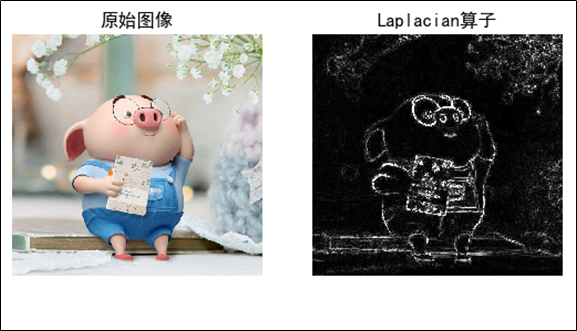# 8 小结

## 8.1 各类算子实验比较

# -*- coding: utf-8 -*-
import cv2
import numpy as np
import matplotlib.pyplot as plt

#读取图像
img_RGB = cv2.cvtColor(img, cv2.COLOR_BGR2RGB) #转成RGB 方便后面显示

#灰度化处理图像
grayImage = cv2.cvtColor(img, cv2.COLOR_BGR2GRAY)

#高斯滤波
gaussianBlur = cv2.GaussianBlur(grayImage, (3,3), 0)

#阈值处理
ret, binary = cv2.threshold(gaussianBlur, 127, 255, cv2.THRESH_BINARY)

#Roberts算子
kernelx = np.array([[-1,0],[0,1]], dtype=int)
kernely = np.array([[0,-1],[1,0]], dtype=int)
x = cv2.filter2D(binary, cv2.CV_16S, kernelx)
y = cv2.filter2D(binary, cv2.CV_16S, kernely)
absX = cv2.convertScaleAbs(x)
absY = cv2.convertScaleAbs(y)
Roberts = cv2.addWeighted(absX, 0.5, absY, 0.5, 0)

#Prewitt算子
kernelx = np.array([[1,1,1],[0,0,0],[-1,-1,-1]], dtype=int)
kernely = np.array([[-1,0,1],[-1,0,1],[-1,0,1]], dtype=int)
x = cv2.filter2D(binary, cv2.CV_16S, kernelx)
y = cv2.filter2D(binary, cv2.CV_16S, kernely)
absX = cv2.convertScaleAbs(x)
absY = cv2.convertScaleAbs(y)

#Sobel算子
x = cv2.Sobel(binary, cv2.CV_16S, 1, 0)
y = cv2.Sobel(binary, cv2.CV_16S, 0, 1)
absX = cv2.convertScaleAbs(x)
absY = cv2.convertScaleAbs(y)
Sobel = cv2.addWeighted(absX, 0.5, absY, 0.5, 0)

#Laplacian算子
dst = cv2.Laplacian(binary, cv2.CV_16S, ksize = 3)
Laplacian = cv2.convertScaleAbs(dst)

# #效果图
# titles = ['Source Image', 'Binary Image', 'Roberts Image',
#           'Prewitt Image','Sobel Image', 'Laplacian Image']
# images = [lenna_img, binary, Roberts, Prewitt, Sobel, Laplacian]
# for i in np.arange(6):
#    plt.subplot(2,3,i+1),plt.imshow(images[i],'gray')
#    plt.title(titles[i])
#    plt.xticks([]),plt.yticks([])
# plt.show()

# 用来正常显示中文标签
plt.rcParams['font.sans-serif'] = ['SimHei']

# # 显示图形
plt.subplot(231),plt.imshow(img_RGB),plt.title('原始图像'), plt.axis('off') #坐标轴关闭
plt.subplot(232),plt.imshow(binary, cmap=plt.cm.gray ),plt.title('二值图'), plt.axis('off')
plt.subplot(233),plt.imshow(Roberts, cmap=plt.cm.gray ),plt.title('Roberts算子'), plt.axis('off')
plt.subplot(234),plt.imshow(Prewitt, cmap=plt.cm.gray ),plt.title('Prewitt算子'), plt.axis('off')
plt.subplot(235),plt.imshow(Sobel, cmap=plt.cm.gray ),plt.title('Sobel算子'), plt.axis('off')
plt.subplot(236),plt.imshow(Laplacian, cmap=plt.cm.gray ),plt.title('Laplacian算子'), plt.axis('off')

plt.show()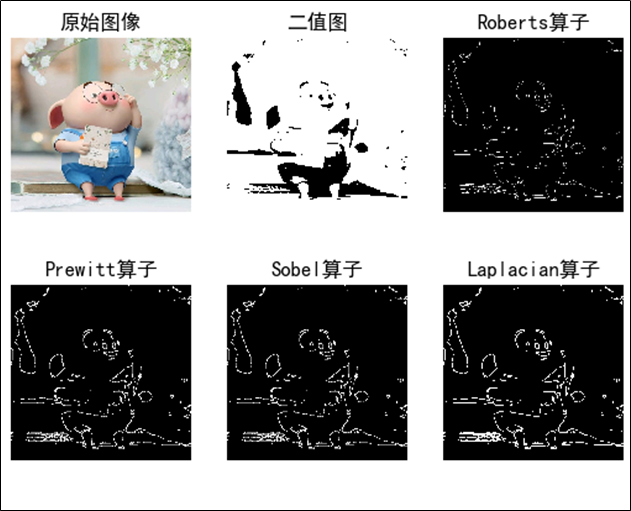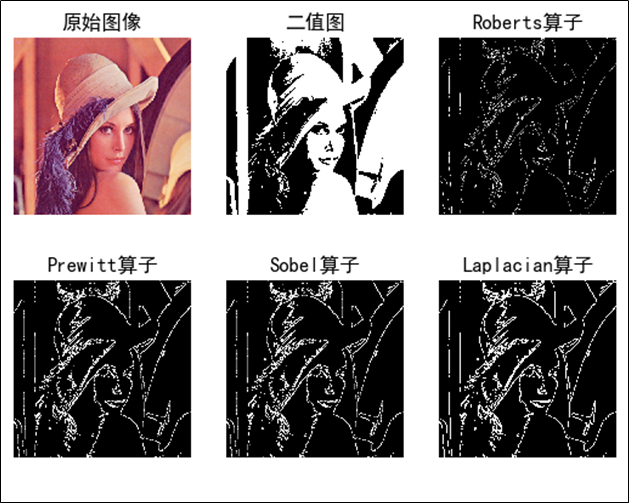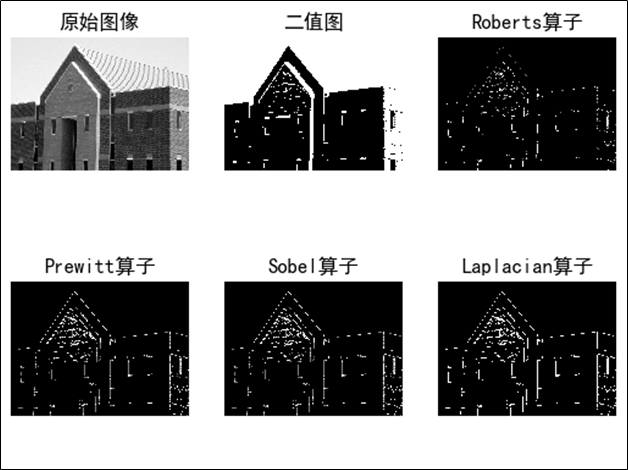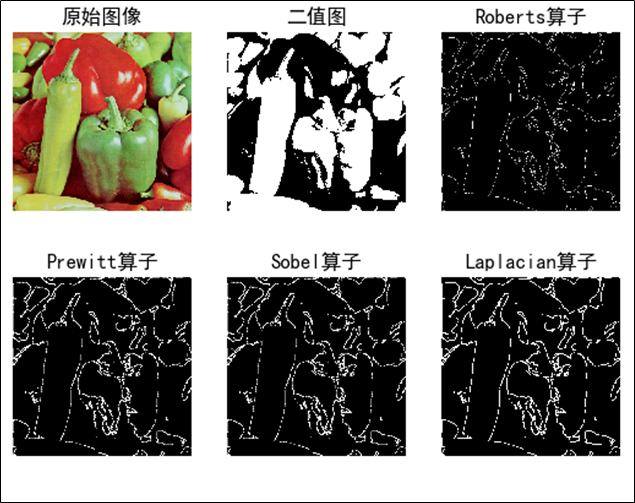1）Robert算子对陡峭的低噪声图像效果较好，尤其是边缘正负45度较多的图像，但定位准确率较差；

2）Prewitt算子对灰度渐变的图像边缘提取效果较好，而没有考虑相邻点的距离远近对当前像素点的影响；

3）Sobel算子考虑了综合因素，对噪声较多的图像处理效果更好。

4）Laplacian算子对噪声比较敏感，由于其算法可能会出现双像素边界，常用来判断边缘像素位于图像的明区或暗区，很少用于边缘检测；

## 8.2 各类算子的优缺点

（1）Roberts 算子

Roberts算子利用局部差分算子寻找边缘，边缘定位精度较高，但容易丢失一部分边缘，不具备抑制噪声的能力。该算子对具有陡峭边缘且含噪声少的图像效果较好，尤其是边缘正负45度较多的图像，但定位准确率较差；

（2）Sobel 算子

Sobel算子考虑了综合因素，对噪声较多的图像处理效果更好，Sobel 算子边缘定位效果不错，但检测出的边缘容易出现多像素宽度。

（3） Prewitt 算子

Prewitt算子对灰度渐变的图像边缘提取效果较好，而没有考虑相邻点的距离远近对当前像素点的影响，与Sobel 算子类似，不同的是在平滑部分的权重大小有些差异；

（4）Laplacian 算子

Laplacian 算子不依赖于边缘方向的二阶微分算子，对图像中的阶跃型边缘点定位准确，该算子对噪声非常敏感，它使噪声成分得到加强，这两个特性使得该算子容易丢失一部分边缘的方向信息，造成一些不连续的检测边缘，同时抗噪声能力比较差，由于其算法可能会出现双像素边界，常用来判断边缘像素位于图像的明区或暗区，很少用于边缘检测；

# 参考资料

 杨帆. 数字图像处理与分析（第三版）. 北京航空航天大学出版社

1. 微信公众号:2. CSDN博客：https://xiongyiming.blog.csdn.net/

10-25334207-047万+
05-031万+
04-181万+
04-032万+
07-08387
10-252万+
04-03462
05-057247
07-114528
©️2020 CSDN 皮肤主题: Age of Ai 设计师:meimeiellie